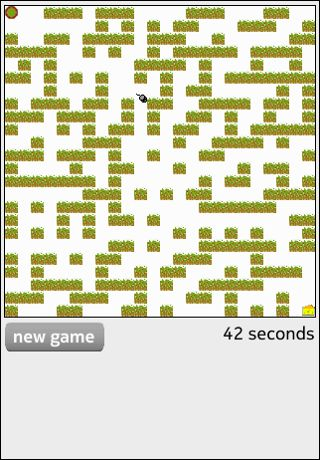# Qt Sensors - Maze QML Example

The Maze example demonstrates the TiltSensor QML type.##### Maze in QML

To write a QML application that will use the TiltSensor QML sensors type you need to do the following steps:

Import the QtSensors 5.x declarative plugin:

```
import QtSensors 5.0
```

Add the Sensor QML types into your qml file.

In this example we use the TiltSensor with values based in degrees and an accuracy of 5 degree:

```
TiltSensor {
id: tiltSensor
active: true
}
```

Starting the sensor can be done by setting the 'enabled' property to true:

```
onTriggered: {
if (!tiltSensor.enabled)
tiltSensor.active = true;
```

The mouse should move by a factor of the tilt value:

```
var xstep = 0;
xstep = tiltSensor.reading.yRotation * 0.1 //acceleration

var ystep = 0;
ystep = tiltSensor.reading.xRotation * 0.1 //acceleration
```

The walk direction of the mouse takes into account some collision detection:

```
if (xstep < 1 && xstep > 0)
xstep = 0
else if (xstep > -1 && xstep < 0)
xstep = 0

if (ystep < 1 && ystep > 0)
ystep = 0;
else if (ystep > -1 && ystep < 0)
ystep = 0;

if ((xstep < 0 && mouseCtrl.x > 0
&& Lib.canMove(mouseCtrl.x + xstep,mouseCtrl.y))) {
xval = mouseCtrl.x + xstep;

} else if (xstep > 0 && mouseCtrl.x < (Lib.cellDimension * (Lib.dimension - 1))
&& Lib.canMove(mouseCtrl.x + xstep,mouseCtrl.y)) {
xval = mouseCtrl.x + xstep;
} else
xval = mouseCtrl.x;

if (ystep < 0 && mouseCtrl.y > 0
&& Lib.canMove(mouseCtrl.x, mouseCtrl.y + ystep)) {
yval = mouseCtrl.y + ystep;
} else if (ystep > 0 && (mouseCtrl.y < (Lib.cellDimension * (Lib.dimension - 1)))
&& Lib.canMove(mouseCtrl.x, mouseCtrl.y + ystep)) {
yval = mouseCtrl.y + ystep;
} else
yval = mouseCtrl.y

mouseCtrl.move(xval, yval);
```

The rotation of the mouse image is determined according to the angle that the mouse is moving.

```
var a = newy - mouse.y
var b = newx - mouse.x
angle = Math.atan2(-b, a) * mouse.radians_to_degrees
if (angle < 0)
angle = 360 + angle

img.rotation = angle
mouse.x = newx;
mouse.y = newy;
```

Example project @ code.qt.io

© 2022 The Qt Company Ltd. Documentation contributions included herein are the copyrights of their respective owners. The documentation provided herein is licensed under the terms of the GNU Free Documentation License version 1.3 as published by the Free Software Foundation. Qt and respective logos are trademarks of The Qt Company Ltd. in Finland and/or other countries worldwide. All other trademarks are property of their respective owners.